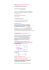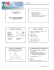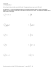# Cascade model - Ewan Colman . Net

## Transcription

Cascade model - Ewan Colman . Net
```Cascade model
Abstract
A model is described in which events happen at discrete time-steps with probability
dependent on the the number of events which occured within some given time interval.
1
Model description
At each discreet time-step the the an agent i is either active (1) or inactive (0). Each
individual is therefore represented by a binary sequence of random variables Xt ∈ 0, 1. The
agent i has an active “memory” which may increase, decrease, or stay the same in each timestep. Suppose i has a memory capacity M , meaning i has M locations m1 (t), m2 (t), ..., mM (t)
where an event may be stored, i.e. mn (t) ∈ 0, 1 for n ∈ 1, 2, ...M . At time t,
P
1. With probability f (kt ), where kt = M
n=1 mn (t), Xt = 1. With probability 1 − f (kt ),
Xt = 0.
2. Integer n0 is selected uniformly at random from 1, 2, ..., M and mn0 (t + 1) = Xt . For
all other n 6= n0 , mn (t + 1) = mn (t).
For the purpose of modelling the inter-event time distribution in social, biological and
physical systems it is necessary to constrain f (k) to the the class of functions which obey
0 < f (k) < 1 for k ∈ 0...M . This avoids the possibility of reaching a terminal state where
Xt = 1 or Xt = 0 for all t > t0 where the concept of inter-event time has little meaning.
1.1
Alternative description
The model given is approximately the same as the following: In each iteration perform step
1 as before, set mM (t + 1) = Xt and mn (t + 1) = mn+1 (t) for 1 ≥ n ≥ M − 1. This way i
will ‘remember’ all the events which happened in the previous M iterations like so:
0000010 1010010
| {z } Xt
M
Assuming that the value of m1 is not correlated with the value of k, i.e. the probability of
removing a 1 is well approximated by k/M , then both descriptions are solved in exactly the
same way.
1
0
1
f (k)
1 − f (k)
k/M
kt+1 = kt
kt+1 = kt − 1
1 − k/M
kt+1 = kt + 1
kt+1 = kt
Probability
Removed
1
0
Table 1: At each iteration Xt ∈ 0, 1 is added to the memory while at the same time a randomly
selected entry will be removed. We show the probabilities of these events and the [effect] this has
on k for each possible combination.
2
Solving for memory size and inter-event time distributions
We first find pk , the probability that kt = k for a randomly selected t ∈ N. For the general
event probability kernel f we find a recursion relation relating pk to pk−1 . We then continue
by examining only the special case where
f (k) =
k+δ
M +δ+
(1)
for constants δ and and find the exact solution for pk . From this result we approximate
the probability that the time between two events is exactly τ iterations of the model.
2.1
Memory size distribution
Table 1 shows the possible events which can happen regarding the addition and deletion of
1s in the memory. All possible transitions of kt are brought together in the following master
equation which describes the evolution of pk (t):
k−1
k
k
pk (t) = 1 −
f (k)pk−1 (t − 1)+ 1 −
− f (k) + 2 f (k) pk (t − 1)
M
M
M
(2)
k+1
+ [1 − f (k + 1)]
pk+1 (t − 1)
M
As t → ∞ the distribution will converge towards a time-invariant distribution, pk , described
by
k−1
k
k
k+1
1−
f (k)pk−1 −
+ f (k) − 2 f (k) pk + [1 − f (k + 1)]
pk+1 = 0. (3)
M
M
M
M
2
This second-order recurrence relation reduces to a first-order recurrence relation with the
introduction of
k
k
[1 − f (k)] pk
and
F (k) = f (k) 1 −
pk ;
(4)
H(k) =
M
M
using the condition that p−1 = 0 in Eq.(3) we see that F (0) = H(1) and also that Eq.(3)
becomes
F (k) − F (k − 1) = H(k + 1) − H(k).
(5)
Clearly then F (k − 1) = H(k), and so pk obeys the more digestable recurrence:
f (k − 1)
M −1−k
pk−1 .
pk =
k
1 − f (k)
(6)
Writing p1 in terms of p0 , then p2 in terms of p1 and so on, we can express Eq.(6) as
k Y
M −1−i
f (i − 1)
pk = p 0
.
i
1
−
f
(i)
i=1
(7)
We choose at this point to investigate only the linear case with f (k) given by Eq.(1). In this
instance the translation property of the Gamma function (xΓ(x) = Γ(x + 1)) can be used
and we arrive at
Γ(M − 1)Γ(M − k + )Γ(k + δ)
pk =
p0
(8)
Γ(M + )Γ(M − k − 1)Γ(δ)k!
giving the probability distribution for the number of 1s in the memory at any given time.
2.2
Inter-event time distribution
Here we derive Πτ , the probability that a randomly selected interval has size τ . Suppose we
select a random Xt from the sequence. For Xt to be 0 and belong to an interval of length τ
it must be preceded by a string which looks like a 1 followed by τ 0 0s, and it must be the first
0 in a sequence of τ − τ 0 0s followed by another 1. The variable τ 0 can be any integer from 1
to τ and summing the probabilities of each possibility we arrive at the probability that Xt is
a 0 at any location within an interval of size τ . This statement is expressed symbolically as
τ Πτ (t) =
τ
X
f (kt−τ 0 )f (kt+τ −τ 0 +1 )
τ 0 =1
t+τ
−τ 0
Y
1 − f (ki )
(9)
i=t−τ 0 +1
where Πτ (t) is the probability that the interval containing Xt has length τ . The multiplication by τ on the left hand side comes from the fact that there are τ choices of Xt which
belong to this interval. We make the following approximations and coarsening of the model:
3
1. We assume that M is large and also consider only the values of k large enough for
Stirling’s approximation to be a valid for the Gamma functions in Eq.(8). We further
limit our attention to those values of k for which M >> k and get
1+
p0 δ−1
p0 δ−1
k
k
≈
k
(10)
pk ≈ 1 −
M
Γ(δ)
Γ(δ)
2. We choose M >> δ, which means f (kt ) ≈ kt /M . More importantly, if we say that
P (f (kt ) = φ) is the probability that f (kt ) = φ for a randomly selected t ∈ N then from
Eq.(10) we have
p0
P (f (kt ) = φ) ≈
[M φ]δ−1 .
(11)
Γ(δ)
3. Over short time periods, changes to kt will be small. In other words, locally the system
behaves as a Bernoulli process with success probability given by φ. This allows Eq.(9)
to be approximated by
Πτ (t) = f (kt )2 [1 − f (kt )]τ .
(12)
When t ∈ N is selected randomly this becomes
P (τ |f (kt ) = φ) = φ2 (1 − φ)τ .
(13)
4. We approximate φ by a continuous variable.
The time-independent solution to the inter-event time distribution is found by solving
Z
Z 1
p0 M δ−1 1 δ+1
φ (1 − φ)τ dφ
(14)
P (φ)P (τ |f (kt ) = φ)dφ ≈
Πτ =
Γ(δ)
0
0
Thus we find that the inter-event time distribution is given by a Beta function Πτ ∼ B(τ +
1, δ + 2). For these probabilities to sum to 1 we divide by the sum over all τ , this gives
Πτ = (δ + 1)B(τ + 1, δ + 2)
(15)
the which for large values of τ obeys
Πτ ∼ τ −(2+δ)
2.3
(16)
Negative values of δ
The event probability kernel given by Eq.(1) is restrictive in that δ must strictly be greater
than 0. One way to broaden the range of δ is to introduce a different transition probability
for when k = 0, i.e.

f0
if k = 0

f (k) =
(17)
k+δ

if k ≥ 1
M +δ+
Provided that f0 > 0 and δ > −1 the condition the 0 < f (k) < M is satisfied. The
calculations of the previous subsections can be repeated for this new kernel and we find that
the results are the same up to multiplication by some constant. Therefore Eq.(16) applies
here.
4
2.4
Inter-event time correlations
Using a similar argument as above we can express the probability of two consecutive interevent times having lengths τ1 and τ2 respectively. The equivalent formula to Eq.(13) is
P (τ1 , τ2 |f (kt ) = φ) = φ3 (1 − φ)τ1 +τ2
(18)
Πτ1 ,τ2 ∼ (τ1 + τ2 )−(3+δ)
(19)
If the two intervals were independent we would expect to find Πτ1 ,τ2 ∼ (τ1 τ2 )−(2+δ) . Since
this is not the case the model predicts a correlation between consecutive intervals. Moreover,
from these formulae we can calculate, and then compare, the likelihood of both models for
any given data set.
5
```

### Chapter 23 Day 1 Objective: Construct confidence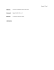### CALCULUS I Worksheet #69 1. Find all inflection points for f(x) = 2x3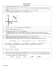### Review SHORT ANSWER. Write the word or phrase that best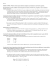### AP Statistics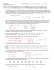### MIDTERM REVIEW SHEET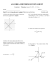### Small-Sample C.I.s for one- sample, two-sample and (matched) paired data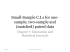### Activity - The IRIS Center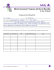### SAMPLE MEANS 8/4/2014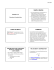### Chapter 7  μ Confidence Intervals and Sample Size